# JP3762579B2 - Digital audio signal encoding apparatus, digital audio signal encoding method, and medium on which digital audio signal encoding program is recorded - Google Patents

Digital audio signal encoding apparatus, digital audio signal encoding method, and medium on which digital audio signal encoding program is recorded Download PDF

## Info

Publication number
JP3762579B2
JP3762579B2 JP22205499A JP22205499A JP3762579B2 JP 3762579 B2 JP3762579 B2 JP 3762579B2 JP 22205499 A JP22205499 A JP 22205499A JP 22205499 A JP22205499 A JP 22205499A JP 3762579 B2 JP3762579 B2 JP 3762579B2
Authority
JP
Japan
Prior art keywords
block
short
acoustic signal
blocks
conversion
Prior art date
Legal status (The legal status is an assumption and is not a legal conclusion. Google has not performed a legal analysis and makes no representation as to the accuracy of the status listed.)
Expired - Fee Related
Application number
JP22205499A
Other languages
Japanese (ja)
Other versions
JP2001053617A (en
Inventor

Original Assignee

Priority date (The priority date is an assumption and is not a legal conclusion. Google has not performed a legal analysis and makes no representation as to the accuracy of the date listed.)
Filing date
Publication date
Application filed by 株式会社リコー filed Critical 株式会社リコー
Priority to JP22205499A priority Critical patent/JP3762579B2/en
Priority claimed from KR1020000045308A external-priority patent/KR100348368B1/en
Publication of JP2001053617A publication Critical patent/JP2001053617A/en
Application granted granted Critical
Publication of JP3762579B2 publication Critical patent/JP3762579B2/en
Anticipated expiration legal-status Critical
Expired - Fee Related legal-status Critical Current

• 238000005070 sampling Methods 0 claims abstract description 15
• 238000006243 chemical reaction Methods 0 claims description 47
• 238000007906 compression Methods 0 claims description 4
• 238000000034 methods Methods 0 description 25
• 230000000873 masking Effects 0 description 14
• 230000000694 effects Effects 0 description 1
• 230000002123 temporal effects Effects 0 description 1

## Images

•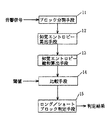•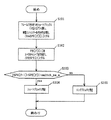•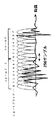••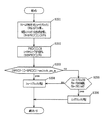•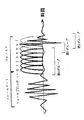•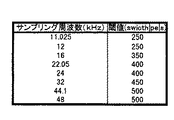••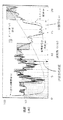•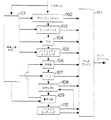•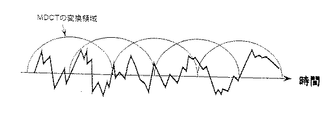•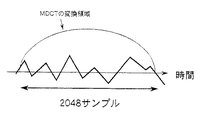•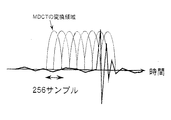•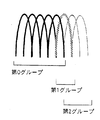•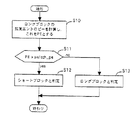•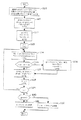••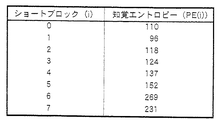•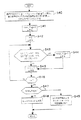## Classifications

• GPHYSICS
• G10MUSICAL INSTRUMENTS; ACOUSTICS
• G10LSPEECH ANALYSIS OR SYNTHESIS; SPEECH RECOGNITION; SPEECH OR VOICE PROCESSING; SPEECH OR AUDIO CODING OR DECODING
• G10L19/00Speech or audio signals analysis-synthesis techniques for redundancy reduction, e.g. in vocoders; Coding or decoding of speech or audio signals, using source filter models or psychoacoustic analysis
• G10L19/02Speech or audio signals analysis-synthesis techniques for redundancy reduction, e.g. in vocoders; Coding or decoding of speech or audio signals, using source filter models or psychoacoustic analysis using spectral analysis, e.g. transform vocoders or subband vocoders
• G10L19/0204Speech or audio signals analysis-synthesis techniques for redundancy reduction, e.g. in vocoders; Coding or decoding of speech or audio signals, using source filter models or psychoacoustic analysis using spectral analysis, e.g. transform vocoders or subband vocoders using subband decomposition
• G10L19/0208Subband vocoders

## Abstract

A digital acoustic signal coding apparatus, a method of coding the digital acoustic signal, and a recording medium for recording a program of coding the digital acoustic signal are respectively realized. It is possible to provide the digital acoustic signal coding method and apparatus, in which, corresponding to the difference between the sampling frequencies of the input acoustic signal, the short blocks can be suitably classified into groups without deteriorating the sound quality and the difference between the long/short blocks can be judged. The coding apparatus is composed of a calculation medium for calculating the sensation entropy of a input acoustic signal calculated per each of the respective short sensation blocks; a sensation entropy sum total calculation medium for obtaining the total sum in the frame of the sensation entropy; a comparison medium for comparing the absolute value of the difference between the respective sum totals in the frame of the sensation entropy of the two frames being successive with a previously determined threshold value; and a long/short block judgment medium for judging whether the long blocks or the short block should convert the block of the input acoustic signal on the basis of the comparison result. <IMAGE>

## Description


BACKGROUND OF THE INVENTION
The present invention relates to a digital audio signal encoding apparatus, a digital audio signal encoding method, and a medium on which a digital audio signal encoding program is recorded, and more particularly to compression / encoding of a digital audio signal used for, for example, a DVD or digital broadcasting.

[Prior art]
Conventionally, human psychoacoustic characteristics have been used in high-quality compression / coding of digital acoustic signals. The characteristic is that a small sound is masked by a loud sound and cannot be heard. That is, when a loud sound is generated at a certain frequency, a sound having a small frequency in the vicinity is masked and cannot be detected by the human ear. Here, the limit intensity that is masked and cannot be heard is called a masking threshold. On the other hand, the human ear has the property that it has the highest sensitivity to sounds near 4 kHz, regardless of masking, and the sensitivity gradually decreases as the band becomes higher and lower than that. This property is expressed as the limit intensity at which sound can be sensed in a quiet situation, which is called the absolute audible threshold.

These will be described with reference to FIG. 9 showing the intensity distribution of the acoustic signal. The thick solid line (A) represents the intensity distribution of the acoustic signal, the dotted line (B) represents the masking threshold for the acoustic signal, and the thin solid line (C) represents the absolute audible threshold. As shown in the figure, the human ear can only detect sounds having an intensity greater than the masking threshold and the absolute audible threshold for an acoustic signal. Therefore, even if only the information of the part larger than the masking threshold value and the absolute audible threshold value for the acoustic signal in the intensity distribution of the acoustic signal is extracted, it can be perceived auditorily as the original acoustic signal.

This is equivalent to assigning coding bits only to the hatched portions in FIG. However, the bit allocation here is performed in units of the divided band (D) by dividing the entire area of the acoustic signal into a plurality of small bands. The horizontal width of each hatched area corresponds to the divided body area width.

In each divided band, sounds below the lower limit intensity of the hatched area cannot be heard. Therefore, the difference between the original sound and the encoded / decoded sound cannot be detected unless the error in intensity exceeds this lower limit. In this sense, the lower limit intensity is called an allowable error intensity. When the sound signal is quantized and compressed, the sound signal can be compressed without degrading the sound quality of the original sound if the quantization error intensity of the encoded / decoded sound with respect to the original sound is quantized to be equal to or less than the allowable error intensity. Therefore, assigning encoded bits only to the shaded area in FIG. 9 is equivalent to performing quantization so that the quantization error intensity in each divided band is just the allowable error intensity.

There are MPEG (Moving Picture Experts Group) Audio, Dolby Digital, and the like as the encoding method of the acoustic signal, and all of them use the properties described here. Among them, a method called MPEG-2 Audio AAC (Advanced Audio Coding) standardized by ISO / IEC 13818-7 is currently considered to have the highest coding efficiency.

FIG. 10 is a block diagram showing a basic configuration of AAC encoding. In the figure, the psychoacoustic model unit 101 calculates an allowable error intensity for each divided band of the input acoustic signal that is blocked along the time axis. On the other hand, the gain control 102 and the filter bank 103 perform conversion into the frequency domain by MDCT (Modified Discrete Cosine Transform), and the TNS (Temporal Noise Shaping) 104 and the predictor 106 predict the same block input signal. Encoding, intensity / coupling 105, and MS stereo (middle side stereo) (hereinafter abbreviated as M / S) 107 perform stereo correlation encoding processing, respectively. Thereafter, the normalization coefficient 108 is determined, and the quantizer 109 quantizes the acoustic signal based on the normalization coefficient 108. This normalization coefficient corresponds to the allowable error intensity of FIG. 9 and is determined for each divided band. After quantization, the noiseless coding 110 performs noiseless coding by giving a Huffman code to each of the normalization coefficient and the quantized value based on a predetermined Huffman code table, and finally forms a code bitstream in the multiplexer 111. .

The MDCT in the above-described filter bank 103 is to perform DCT while overlapping the transform regions by 50% along the time axis as shown in FIG. This suppresses the occurrence of distortion at the boundary between the conversion regions. The number of MDCT coefficients generated is half the number of samples in the transform area. In AAC, either a long conversion region (long block) of 2048 samples or eight short conversion regions (short block) of 256 samples is applied to an input acoustic signal block. Therefore, the number of MDCT coefficients is 1024 for long and 128 for short. The short block always applies 8 blocks in succession, so that the number of MDCT coefficients is matched with the case of using the long block.

In general, a long block is used for a stationary part where the change in signal waveform is small as shown in FIG. 12, and a short block is used for an attack part where change is rapid as shown in FIG. The use of both is important. If a long block is applied to a signal as shown in FIG. 13, noise called pre-echo occurs before the original attack. In addition, when a short block is applied to a signal as shown in FIG. 12, encoding efficiency is lowered without appropriate bit allocation due to insufficient resolution in the frequency domain, noise is generated, and particularly low frequency sound is generated. This is remarkable.

There is a further grouping problem with short blocks. Grouping means that the above eight short blocks are grouped together for each consecutive block having the same normalization coefficient. By sharing the normalization coefficient within the group, the information amount can be reduced. Specifically, when the Huffman code is assigned to the normalization coefficient in the noiseless coding 110 of FIG. 10, it is assigned in units of groups rather than in units of short blocks. FIG. 14 shows an example of grouping. Here, the number of groups is 3, and the number of blocks in each group is 5 for the first group 0, 1 for the next group 1, and 2 for the last group 2. If the grouping is not performed appropriately, the amount of code increases and the sound quality deteriorates. If the number of group divisions is too large, normalization coefficients that should be able to be shared in common will be encoded redundantly, resulting in a decrease in encoding efficiency. On the other hand, if the number of groups is too small, the sound quality deteriorates because quantization is performed with a common normalization coefficient even though the acoustic signal changes drastically. In ISO / IEC13818-7, although there is a code syntax rule regarding grouping, specific grouping standards and methods are not considered.

As described above, in encoding, a long block and a short block must be appropriately distinguished and applied to an input audio signal block. The auditory psychological model unit 101 in FIG. 10 performs the long / short determination. ISO / IEC13818-7 describes an example of a long / short determination method for each block of interest in the psychoacoustic model unit 101. An outline of the determination process will be described below.

Step 1: Reconstruct sound signal
Newly read 1024 samples for the long block (128 samples for the short block), and reconstruct the signal sequence of 2048 samples (256 samples) together with the 1024 samples (128 samples) already read in the previous block .

Step 2: Han window crossing and FFT
The acoustic signal of 2048 samples (256 samples) constructed in Step 1 is multiplied by a Hann window and further subjected to FFT (Fast Fourier Transform) to calculate 1024 (128) FFT coefficients.

Step 3: Calculate the predicted value of the FFT coefficient
The real part and imaginary part of the FFT coefficient of the currently focused block are predicted from the real part and imaginary part of the FFT coefficients for the two preceding blocks, and 1024 (128) predicted values are calculated.

Step 4: Calculate non-predictability values
Each non-predictability value is calculated from the real part and imaginary part of each FFT coefficient calculated in step 2 and the predicted values of the real part and imaginary part of each FFT coefficient calculated in step 3. Here, the non-predictability value takes a value between 0 and 1, and the closer to 0, the higher the pure tone property of the acoustic signal, and the closer to 1, the higher the noise property, in other words, the lower the pure tone property.

Step 5: Calculation of the intensity and non-predictability value of the acoustic signal in each divided band
The divided bands here correspond to those shown in FIG. For each divided band, the intensity of the acoustic signal is calculated based on each FFT coefficient calculated in step 2. Further, the non-predictability value calculated in step 4 is weighted by intensity, and the non-predictability value for each divided band is calculated.

Step 6: Convolution of intensity multiplied by spread function and non-predictability value
The influence of the acoustic signal intensity and the non-predictability value in the other divided bands in each divided band is obtained by a spread function, and each is convolved and normalized.

Step 7: Calculation of pure tone index
In each divided band b, based on the convolutional non-predictability value (cb (b)) calculated in step 6, the pure tone index tb (b) (= −0.299−0.43 log) e (Cb (b))) is calculated. Further, the pure tone index is limited between 0 and 1. Here, the closer the index is to 1, the higher the pure tone property of the acoustic signal, and the closer to 0, the higher the noise property.

Step 8: Calculation of S / N ratio
In each divided band, the S / N ratio is calculated based on the pure tone index calculated in step 7. Here, the characteristic that the noise component generally has a larger masking effect than the pure tone component is utilized.

Step 9: Calculation of intensity ratio
In each divided band, the ratio between the convolutional sound signal intensity and the masking threshold is calculated based on the S / N ratio calculated in step 8.

Step 10: Calculation of allowable error intensity
In each divided band, the masking threshold is calculated based on the convolutional sound signal intensity calculated in Step 6 and the ratio of the sound signal intensity calculated in Step 9 and the masking threshold.

Step 11: Consider pre-echo adjustment and absolute audible threshold
In each divided band, the pre-echo adjustment is performed on the masking threshold calculated in step 10 using the allowable error intensity in the previous block. Further, the larger value of the adjustment value and the absolute audible threshold is set as the allowable error intensity in the current block.

Step 12: Calculate perceptual entropy
The perceptual entropy (Perceptual Entropy (PE)) defined by Equation (1) is calculated for each of the long block and the short block.

[Expression 1]

Where w (b) is the width of the divided band b, nb (b) is the allowable error intensity in the divided band b calculated in step 11, and e (b) is the acoustic signal in the divided band b calculated in step 5. Strength. Here, the PE is considered to correspond to the total area of the bit allocation areas (shaded areas) in FIG.

Step 13: Long / short block determination (see long / short block determination operation flow shown in FIG. 15)
If the PE value for the long block calculated in step 12 (step S10) is larger than a predetermined constant (switch_pe), the target block is determined to be a short block (steps S11 and S12), and if it is smaller, It is determined that the block is a long block (steps S11 and S13). Here, switch_pe is a value determined depending on the application.

The above is the long / short determination method described in ISO / IEC13818-7. However, the long / short block determination method described in the above-mentioned ISO / IEC13818-7 does not always make an appropriate determination. In other words, a portion that should be determined to be short may be determined to be long (or vice versa) and sound quality may be degraded.

On the other hand, in Japanese Patent Application Laid-Open No. 9-232964, an input signal is taken every predetermined interval to obtain a sum of squares, and the above signal is determined according to the degree of change over at least two or more intervals of the sum of squares for each interval. The transient state detection circuit 2 is configured so as to detect the transient state, and the transient state, that is, long / short, is simply performed by calculating the sum of squares of the input signal on the time axis without performing the orthogonal transformation process and the filter process. It is made possible to detect the part where changes. In this method, only the sum of squares of the input signal is used and perceptual entropy is not taken into consideration, so that it is not always possible to make a determination that matches auditory characteristics, and sound quality may be deteriorated.

Therefore, the input acoustic signal blocks are grouped so that the difference between the maximum and minimum perceptual entropy values for each short block in the same group is smaller than a predetermined threshold. As a result, when the number of groups is 1, Alternatively, when this and other conditions are satisfied, there is a method in which the input acoustic signal block is converted into the frequency domain by one long block, and in other cases, the input acoustic signal block is converted by a plurality of short blocks. This method will be described below with reference to FIG. As an example of the input sound signal, the sound data of FIG. 17 is used, and in FIG. 17, serial numbers corresponding to eight consecutive short blocks are given.

First, the input acoustic signal is divided into eight continuous short blocks. Then, the perceptual entropy of each of the eight short blocks is calculated, and these are sequentially set to PE (i) (0 ≦ i ≦ 7) (step S20). This calculation is realized by performing, for each short block, the method described in step 1 to step 12 of the long / short determination method for each block of interest in the above-mentioned ISO / IEC13818-7. Next, it is initialized as group_len  = 1, group_len [gnum] = 0 (0 ≦ gnum ≦ 7) (step S21). Here, gnum represents the group serial number in the group, and group_len [gnum] represents the number of short blocks included in the gnum group. Then, gnum = 0, min = PE (0), and max = PE (0) are initialized (step S22). The min and max represent the minimum value and the maximum value of PE (i), respectively. According to FIG. 18, here, min = 110 and max = 110. Further, the index i is initialized to i = 1 (step S23). This index corresponds to the serial number of the short block.

Next, min or max is updated by PE (i). That is, if PE (i) <min, min = PE (i), and if PE (i)> max, max = PE (i) (step S24). In the example of FIG. 18, since PE (1) = 96, min = 96 and max = 110. Then, grouping is determined (step S25). That is, when the obtained max-min is compared with a predetermined threshold th and is equal to or greater than the threshold th, the process proceeds to step S26 to perform grouping between the short blocks i-1 and i, and is smaller than th. In this case, it is determined that the short blocks i-1 and i are included in the same group, and the process proceeds to step S27. In this example, th = 50 is set. That is, grouping is performed so that the difference between the maximum value and the minimum value of PE (i) of each short block included in the same group is smaller than 50. When i = 1, since max−min = 110−96 = 14 <50 = th, it is determined that short blocks 0 and 1 are included in the same group, and the process proceeds to step S27. Since gnum = 0 here, short blocks 0 and 1 are included in the 0th group. Then, the value of group_len [gnum] is incremented by 1 (step S28). This means that the number of short blocks included in the gnum group is increased by one. In this example, since gnum = 0 and group_len  = 1 are initialized in steps S21 and S22, group_len  = 2 in step S27. This corresponds to the fact that two blocks 0 and 1 are already determined as short blocks included in the 0th group.

Next, the index i is incremented by 1 (step S28), and if i is smaller than 7, the process returns to step S24 (step S29). In this example, since i = 2 <7, the process returns to step S24.

Thereafter, the same operation as described above continues until i = 4. When i = 4, as shown in FIG. 18, since min = 96 and max = 137 in step S24 of FIG. 16, it is determined in step S25 that max−min = 41 <50 = th, and again from step S25. The process proceeds to step S27 as it is. In step S27, group_len  = 5. This corresponds to the fact that five blocks 0, 1, 2, 3 and 4 are determined as short blocks included in the 0th group. Then, after i = 5 in step S28, when returning to step S24 again through step S29, this time, PE (5) = 152, so min = 96 and max = 152. Then, since it is determined in step S25 that max-min = 56> 50 = th, the process proceeds to step S26. This means that grouping is performed between the short blocks 4 and 5. In step S26, the value of gnum is incremented by 1, and min and max are replaced with the latest PE (i), respectively. Here, gnum = 1, min = 152, and max = 152. gnum = 1 corresponds to the fact that the group including the short block 5 is the first group.

Next, group_len  is incremented by 1 in step S27. Since group_len  has been initialized to 0 in step S21, group_len  = 1 again. This corresponds to the determination of one block of the block 5 as a short block included in the first group.

Similarly, when i = 6 in step S28 of FIG. 16 and returning to step S24 from step S29, PE (6) = 269 from FIG. 18 this time, min = 152, max = 269, and step S85 follows. Therefore, it is determined that max−min = 117> 50, and the process proceeds to step S26. That is, grouping is also performed between the short blocks 5 and 6. In step S26, gnum = 2, min = 269, and max = 269, and in step S27, group_len  = 1. Then, after i = 7 in step S28, PE (7) = 231 in step S24, so min = 231 and max = 269, and in step S25, it is determined that max−min = 38 <50. Then, the process proceeds to step S27. That is, both the short blocks 6 and 7 are included in the second group. Correspondingly, group_len  = 2 in step S27. When i = 8 in the next step S28, the process proceeds to step S30 based on the determination in step S29. This completes grouping for all eight short blocks.

In this example, gnum = 2, group_len  = 5, group_len  = 1, and group_len  = 2. That is, the number of groups is 3, and the number of short blocks included in each group is 5 for the 0th group, 1 for the first group, and 2 for the second group. This is the same as the grouping example shown in FIG.

However, there are cases where it is not possible to make an appropriate long / short determination even with this method. In some cases, acoustic data including a component having a high pure tone in a low frequency component is encoded. While conversion by a short block increases the resolution in the time domain, the resolution in the frequency domain decreases. On the other hand, the human ear has a high-resolution masking characteristic in a low frequency region, and only a very narrow frequency band is masked particularly for acoustic data with a high pure tone.

However, when acoustic data containing a low-frequency component with a high-pure tone component is converted with a short block, the energy of the original acoustic data is dispersed in the surrounding frequency bands due to insufficient resolution in the frequency domain caused by the short block. As a result, it spreads beyond the masking range of the low-frequency component of the human ear, and as a result, the sound quality deteriorates. This means that it is not sufficient to make a long / short determination based solely on the perceptual entropy of the short block, and it is necessary to consider a combination of the pure tone of the acoustic data and the frequency dependence of the masking characteristics. It is shown that.

Therefore, we next divide the input acoustic signal frame into a plurality of short blocks, and for each short block, the pure tone index of the acoustic component included in one or more predetermined sub-bands is determined for each sub-band. In the case where there is at least one short block whose pure tone index is larger than the predetermined threshold in all of the predetermined one or a plurality of divided bands. Filed a method for determining that an input acoustic signal frame should be converted into the frequency domain in one long block. FIG. 19 is a flowchart showing a specific implementation example of this method.

FIG. 19 is a flowchart showing the operation of the digital audio signal encoding apparatus. Hereinafter, the specific operation of the present embodiment will be described with reference to both drawings. At this time, the acoustic data of FIG. 17 is used as an example of the input acoustic signal. In FIG. 17, serial numbers corresponding to eight consecutive short blocks are given.

First, regarding the input acoustic signal, the pure tone index in each divided band sfb is calculated for eight consecutive short blocks i (0 ≦ i ≦ 7), and these are set as tb [i] [sfb] ( Step S40). Here, sfb is a serial number for identifying each divided band as shown in FIG. The pure tone index is calculated by the method described in step 7 of the long / short determination steps for each block of interest in the ISO / IEC13818-7. Next, initialization is performed with tonal_flag = 0 (step S41). Further, the serial number i of the short block is initialized to i = 0 (step S42). Then, regarding the short block i, it is checked whether or not each pure tone index is larger than a predetermined threshold value for each divided band in one or more predetermined divided regions (step S43). In the example of FIG. 19, the divided areas where sfb = 7, 8, and 9 are examined, and the threshold values of the pure tone index are set to th7, th8, and th9, respectively.

In the example here, for each short block i, it is assumed that the value of the pure tone index at stb = 7, 8, 9 is as shown in FIG. Further, it is assumed that th7 = 0.6, th8 = 0.9, and th9 = 0.8. Then, when the first i = 0, tb   = 0.12 <0.6 = th7, tb pre   = 0.08 <0.9 = th8, tb [ Since 0]  = 0.15 <0.8 = th9, the determination in step S43 is no, and the process proceeds to the next step S106. Then, the value of i is incremented by one to become i = 1, and after the determination in step S46, the process returns to step S43 again.

Thereafter, the same operation as described above continues until i = 5. After i = 6 (step S45), the process returns to step S43 again through step S46. From now on, tb   = 0.67> 0.6 = th7, tb   = 0.95> 0.9 = th8, tb   = 0.89 Since> 0.8 = th9, the determination in step S43 is yes, and the process proceeds to step S44. Then, tonal_flag = 1 is set (step S44). Next, i = 7 (step S45), and the process returns to step S43 through step S46. For i = 7, tb   = 0.42 <0.6 = th7, tb   = 0.84 <0.9 = th8, tb   = 0. Since 81> 0.8 = th9, the determination in step S43 is no, and the process proceeds to step S45. On the other hand, tonal_flag = 1 remains unchanged. After i = 8 (step S45), the process proceeds to step S47 through the determination of step S46. Then, the value of tonal_flag is checked (step S47). In this example, since tonal_flag = 1, the determination is yes and the process proceeds to step S48. Therefore, it is determined that the input acoustic block is subjected to MDCT conversion by one long block.

[Problems to be solved by the invention]
However, even with these methods, there is a case where the long / short determination is not properly performed. Although the conversion is normally performed with a short block, the result of grouping according to the conventional example is one group, so that it may be determined as a long block. Further, according to FIG. 9, the contribution of the absolute audible threshold decreases as the sampling frequency of the input acoustic signal decreases in the region of 4 kHz or higher, so the area of the bit allocation region (hatched region in FIG. 9) is relatively To increase. As a result, the perceptual entropy (PE) value calculated in step 12 in the long / short block determination method described in the above-mentioned ISO / IEC13818-7 also increases. However, if the threshold regarding the difference in the total value of the perceptual entropy of each short block is a common value regardless of the sampling frequency, even if a long / short can be determined appropriately at a certain sampling frequency, In such a case, there arises a problem that proper determination cannot be made.

The present invention is for solving these problems, and in response to the difference in the sampling frequency of the input acoustic signal, the short blocks are appropriately grouped so that the sound quality does not deteriorate, and the long / short type is also obtained. It is an object of the present invention to provide a digital audio signal device, a digital audio signal encoding method, and a medium on which a digital audio signal encoding program is recorded.

[Means for Solving the Problems]
In order to solve the above problems, the present invention provides a perceptual entropy calculating means for calculating perceptual entropy of an input acoustic signal calculated for each short transform block, and a perceptual entropy calculating means for calculating the perceptual entropy within the frame of the perceptual entropy calculating means. Perceptual entropy sum calculating means for obtaining the sum of the two, comparison means for comparing the absolute value of the difference between the sums of the perceptual entropy of two consecutive frames in time with a predetermined threshold, and comparing means And a long / short block determining means for determining whether to convert the block of the input sound signal as a long block or a short block based on the comparison result of the above. The long / short block determination means determines that the temporally subsequent frame of two consecutive frames in time is converted by the short block when the absolute value is larger than the threshold value in the comparison result by the comparison means, and is smaller. It is determined that a temporally subsequent frame of two temporally continuous frames is converted by a long block. Therefore, it is possible to provide a digital audio signal encoding device capable of determining whether the input signal is long or short according to the characteristics of the input audio signal.

As another invention, perceptual entropy calculating means for calculating perceptual entropy of the input acoustic signal calculated for each short transform block, and perception for calculating the sum of the perceptual entropy calculated by the perceptual entropy calculating means within the frame. The entropy sum calculation means, the comparison means for comparing the absolute value of the difference between the sums of the frames in the perceptual entropy of two temporally continuous frames with a predetermined threshold, and the comparison result by the comparison means are absolute. When the value is larger than the threshold value, it is characterized in that it comprises determination means for determining that a temporally subsequent frame is converted by a short block among two temporally consecutive frames, and determining that determination is impossible when the value is smaller. Therefore, it is possible to provide a digital audio signal encoding apparatus that can perform block conversion determination that further reflects the characteristics of the input audio signal.

Furthermore, by determining the threshold value for each sampling frequency of the input sound signal, it is possible to determine whether the long / short is appropriate according to the difference in the sampling frequency of the input sound signal.

In another digital audio signal encoding method according to another invention, the perceptual entropy of the input acoustic signal calculated for each short transform block is calculated, and the sum of the calculated perceptual entropy within the frame is obtained. The absolute value of the difference between the sums of the perceptual entropy of two consecutive frames in the frame is compared with a predetermined threshold, and the block of the input acoustic signal is either a long block or a short block based on the comparison result To determine whether to convert. In addition, when the block of the input acoustic signal is converted to either the long block or the short block, when the absolute value is larger than the threshold, the temporally subsequent frame of the two temporally consecutive frames is a short block. If it is small, it is determined that a frame that is temporally later of two frames that are temporally continuous is converted by a long block. Therefore, it is possible to provide a digital audio signal encoding method capable of determining long / short according to the characteristics of the input audio signal.

Another digital audio signal encoding method calculates the perceptual entropy of the input audio signal calculated for each short transform block, obtains the sum of the calculated perceptual entropy within the frame, and is continuous in time. The absolute value of the difference between the sums in the perceptual entropy of the two frames is compared with a predetermined threshold value. If the absolute value is greater than the threshold value, the two temporally consecutive frames are later in time. It is determined that the frame is converted with a short block. Therefore, it is possible to provide a digital audio signal encoding method capable of performing block conversion determination that further reflects the characteristics of the input audio signal.

Furthermore, by using a medium recorded with a program for executing the digital audio signal encoding method of the present invention, an apparatus for constructing an encoding system can be used universally without changing an existing system.

DETAILED DESCRIPTION OF THE INVENTION
Perceptual entropy calculating means for calculating the perceptual entropy of the input acoustic signal calculated for each short transform block, perceptual entropy sum calculating means for calculating the sum of the perceptual entropy calculated by the perceptual entropy calculating means in the frame, and time A comparison means for comparing the absolute value of the difference between the sum of the perceptual entropy of two consecutive frames in a frame with a predetermined threshold, and a block of the input acoustic signal based on the comparison result by the comparison means And a long / short block determining means for determining whether to convert a block with a long block or a short block.

【Example】
Embodiments of the present invention will be described below with reference to the drawings.
FIG. 1 is a block diagram showing the configuration of a digital audio signal encoding apparatus according to an embodiment of the present invention. The digital audio signal encoding apparatus of the present embodiment shown in the figure includes a block dividing unit 11 that divides an inputted audio signal into a predetermined number, in the following description, eight continuous blocks, and perception of each divided block. Perceptual entropy calculating means 12 for calculating the entropy PE by the above-described calculation formula, perceptual entropy sum calculating means 13 for calculating the sum of the calculated perceptual entropy within the frame, within the frame of the perceptual entropy of two temporally continuous frames Comparing means 14 for comparing the absolute value of the difference between each sum and a predetermined threshold value, and long / short block determining means 15 for determining either a long block or a short block according to the comparison result. ing.

FIG. 2 is a flowchart showing the operation of the digital audio signal encoding apparatus according to the first embodiment of the present invention. Hereinafter, the specific operation of the present embodiment will be described with reference to both drawings. At this time, the acoustic data of FIG. 3 is used as an example of the input acoustic signal. Here, a total of 16 short blocks included in two temporally continuous frames are shown. The frames are frame f-1 and frame f in time order, and the frame currently focused on is the later frame f. Further, a serial number corresponding to each short block is given in each frame.

First, perceptual entropy calculating means 12 calculates perceptual entropy PE [f] [i] for each of eight consecutive short blocks i (0 ≦ i ≦ 7) in frame f by block dividing means 11 (step S101). ). This perceptual entropy is calculated by the method described in step 12 in the long / short block determination method described in the above-mentioned ISO / IEC13818-7. Next, the total value SPE [f] related to 0 ≦ i ≦ 7 of PE [f] [i] is obtained by the perceptual entropy sum calculating means 13 as defined by the following equation (step S102).

[Expression 2]

Then, the absolute value of the difference between SPE [f−1] and SPE [f] that has already been obtained in the same manner as described above in the previous frame f−1 is obtained by the comparison means 14, and the predetermined threshold value switch_pe_s Are compared (step S103). The long / short block determining means 15 proceeds to step S104 if it is larger than switch_pe_s, and determines that the frame f is converted by a plurality of short blocks. On the other hand, when it is smaller than switch_pe_s, the process proceeds to step S105, and it is determined that the frame f is converted by one long block.

FIG. 4 is a diagram showing PE [f] [i] corresponding to each short block in FIG. In the example shown in the figure, since SPE [f−1] = 1390 and SPE [f] = 1030, when switch_pe_s = 500, | SPE [f−1] −SPE [f] | = 360 <switch_pe_s = Therefore, it is determined that the frame f is converted by one long block.

Next, the operation of the digital audio signal encoding apparatus according to the second embodiment of the present invention will be described with reference to the flowchart shown in FIG. Note that steps S201 to S204 are the same as steps S101 to S104 in FIG. 2, and different operations will be described. In step S203, the absolute value of the difference between SPE [f-1] and SPE [f] that has already been obtained by the same method as described above in the previous frame f-1 is obtained, and the magnitude of the difference between the absolute value and a predetermined threshold value switch_pe_s. Compare If it is larger than switch_pe_s, the process proceeds to step S204, and it is determined that the frame f is converted by a plurality of short blocks. On the other hand, if it is smaller than switch_pe_s, the process proceeds to step S205, where it is impossible to determine only by the difference information of the total value of the perceptual entropy of each short block in the frame, and long / short is determined by other means. As an example, the frames f are grouped so that the difference between the maximum and minimum perceptual entropy values for each short block in the same group is smaller than a predetermined threshold value. In step S206, it is determined that the frame f is converted into the frequency domain with one long block, and in other cases, it is determined that the process proceeds to step S204 and is converted with a plurality of short blocks. The details of the grouping are as shown in the flowchart of FIG.

As a specific example, let us consider an example including FIG. 6 showing the result of grouping frames f in addition to FIGS. 3 and 4. Also here, switch_pe_s = 500. As described above, in the example shown in FIG. 3 and FIG. 4, | SPE [f-1] −SPE [f] | = 360 <switch_pe_s = 500, so it is finally left to the determination based on the grouping result. In FIG. 6, frames f are grouped into three groups (short blocks i = 0, 1, 2, 3, and 4 are the 0th group, i = 5 is the 1st group, and i = 6 and 7 are the 2nd group. Therefore, it is determined that conversion is performed using a plurality of short blocks. The long / short determination method used in step S205 is not limited to the method based on the grouping result used here, and other determination methods may be used. 2 and FIG. 5, one switch_pe_s is determined. However, as shown in FIG. 7 showing an example of the value of switch_pe_s for each sampling frequency, it is determined for each sampling frequency of the input acoustic signal, and the actually input sound The value of switch_pe_s may be set according to the sampling frequency of the signal with reference to FIG.

Next, FIG. 8 is a block diagram showing the system configuration of the present invention. That is, this figure shows hardware constructed from a microprocessor or the like that executes software according to the digital audio signal encoding method in the above embodiment. In the figure, the digital audio signal encoding system includes an interface (hereinafter abbreviated as I / F) 81, a CPU 82, a ROM 83, a RAM 84, a display device 85, a hard disk 86, a keyboard 87, and a CD-ROM drive 88. . A general-purpose processing device is prepared, and a program for executing the digital audio signal encoding method of the present invention is recorded on a readable recording medium such as a CD-ROM 89. Furthermore, a control signal is input from an external device via the I / F 81, and an instruction from the operator or a program of the present invention is automatically activated by the keyboard 87. Then, the CPU 82 performs encoding control processing according to the above-described digital audio signal encoding method according to the program, stores the processing result in a storage device such as the RAM 84 or the hard disk 86, and outputs it to the display device 85 or the like as necessary. As described above, by using the medium recorded with the program for executing the digital audio signal encoding method of the present invention, the apparatus for constructing the encoding system can be used universally without changing the existing system. Can do.

In addition, this invention is not limited to the said Example, It cannot be overemphasized that various deformation | transformation and substitution are possible if it is described in a claim.

【The invention's effect】
As described above, according to the present invention, the perceptual entropy calculating means for calculating the perceptual entropy of the input acoustic signal calculated for each short transform block, and the perceptual entropy calculating means calculated by the perceptual entropy calculating means Perceptual entropy sum calculating means for obtaining the sum of the two, comparison means for comparing the absolute value of the difference between the sums of the perceptual entropy of two consecutive frames in time with a predetermined threshold, and comparing means And a long / short block determining means for determining whether to convert the block of the input sound signal as a long block or a short block based on the comparison result of the above. The long / short block determination means determines that the temporally subsequent frame of two consecutive frames in time is converted by the short block when the absolute value is larger than the threshold value in the comparison result by the comparison means, and is smaller. It is determined that a temporally subsequent frame of two temporally continuous frames is converted by a long block. Therefore, it is possible to provide a digital audio signal encoding device capable of determining whether the input signal is long or short according to the characteristics of the input audio signal.

As another invention, perceptual entropy calculating means for calculating perceptual entropy of the input acoustic signal calculated for each short transform block, and perception for calculating the sum of the perceptual entropy calculated by the perceptual entropy calculating means within the frame. The entropy sum calculation means, the comparison means for comparing the absolute value of the difference between the sums of the frames in the perceptual entropy of two temporally continuous frames with a predetermined threshold, and the comparison result by the comparison means are absolute. When the value is larger than the threshold value, it is characterized in that it comprises determination means for determining that a temporally subsequent frame is converted by a short block among two temporally consecutive frames, and determining that determination is impossible when the value is smaller. Therefore, it is possible to provide a digital audio signal encoding apparatus that can perform block conversion determination that further reflects the characteristics of the input audio signal.

Furthermore, by determining the threshold value for each sampling frequency of the input sound signal, it is possible to determine whether the long / short is appropriate according to the difference in the sampling frequency of the input sound signal.

In another digital audio signal encoding method according to another invention, the perceptual entropy of the input acoustic signal calculated for each short transform block is calculated, and the sum of the calculated perceptual entropy within the frame is obtained. The absolute value of the difference between the sums of the perceptual entropy of two consecutive frames in the frame is compared with a predetermined threshold, and the block of the input acoustic signal is either a long block or a short block based on the comparison result To determine whether to convert. In addition, when the block of the input acoustic signal is converted to either the long block or the short block, when the absolute value is larger than the threshold, the temporally subsequent frame of the two temporally consecutive frames is a short block. If it is small, it is determined that a frame that is temporally later of two frames that are temporally continuous is converted by a long block. Therefore, it is possible to provide a digital audio signal encoding method capable of determining long / short according to the characteristics of the input audio signal.

Another digital audio signal encoding method calculates the perceptual entropy of the input audio signal calculated for each short transform block, obtains the sum of the calculated perceptual entropy within the frame, and is continuous in time. The absolute value of the difference between the sums in the perceptual entropy of the two frames is compared with a predetermined threshold value. If the absolute value is greater than the threshold value, the two temporally consecutive frames are later in time. It is determined that the frame is converted with a short block. Therefore, it is possible to provide a digital audio signal encoding method capable of performing block conversion determination that further reflects the characteristics of the input audio signal.

Furthermore, by using a medium recorded with a program for executing the digital audio signal encoding method of the present invention, an apparatus for constructing an encoding system can be used universally without changing an existing system.
[Brief description of the drawings]
FIG. 1 is a block diagram showing a configuration of a digital audio signal encoding device according to the present invention.
FIG. 2 is a flowchart showing the operation of the digital audio signal encoding method according to the first embodiment of the present invention.
FIG. 3 is a diagram illustrating a signal waveform of an example of an acoustic signal in the first embodiment.
FIG. 4 is a diagram illustrating a relationship between perceptual entropy values in two temporally continuous frames for each short block.
FIG. 5 is a flowchart showing the operation of the digital audio signal encoding method according to the second embodiment of the present invention.
FIG. 6 is a diagram showing an example of grouping in the second embodiment.
FIG. 7 is a diagram illustrating an example of a threshold value for each sampling frequency.
FIG. 8 is a block diagram showing a system configuration of the present invention.
FIG. 9 is a diagram showing intensity distributions of an acoustic signal, a masking threshold value, and an absolute audible threshold value.
FIG. 10 is a block diagram showing a basic configuration of AAC encoding.
FIG. 11 is a diagram illustrating a conversion region of MDCT.
FIG. 12 is a diagram showing a conversion region of MDCT in the case of a signal waveform with little change.
FIG. 13 is a diagram showing a conversion region of MDCT in the case of a signal waveform that changes drastically.
FIG. 14 is a diagram illustrating an example of grouping.
FIG. 15 is a flowchart showing a long / short block determination operation in ISO / IEC13818-7.
FIG. 16 is a flowchart showing the operation of a conventional digital audio signal encoding method.
FIG. 17 is a diagram illustrating a signal waveform of an example of an acoustic signal.
FIG. 18 is a diagram showing a relationship with perceptual entropy for a short block.
FIG. 19 is a flowchart showing the operation of another conventional digital audio signal encoding method.
[Explanation of symbols]
11 block dividing means, 12 perceptual entropy calculating means,
13 perceptual entropy sum calculating means, 14 comparing means,
15 long / short block judging means, 81 I / F, 82 CPU,
83 ROM, 84 RAM, 85 display device, 86 hard disk,
87 keyboard, 88 CD-ROM drive, 89 CD-ROM.

## Claims (10)

1. Digital sound signals are input along the time axis to be divided into blocks, and each block is subjected to processing such as subband division and conversion to the frequency domain, and the sound signals are divided into a plurality of bands. A digital acoustic signal encoding apparatus that allocates normalized bits, obtains a normalization coefficient according to the number of allocated encoded bits, and performs compression encoding by quantizing the acoustic signal with the normalization coefficient, the frequency When performing conversion to a region, the acoustic signal that has been blocked is converted by either one long conversion block or a plurality of short conversion blocks, and when using a short conversion block, the plurality of short conversion blocks, Group into multiple blocks, each containing one or more short transform blocks, into one or more short transform blocks contained in the same group In the digital acoustic signal encoding apparatus for quantizing audio signals in correspondence a common normalization factor,
Perceptual entropy calculating means for calculating perceptual entropy of the input acoustic signal calculated for each short transform block;
Perceptual entropy sum calculating means for calculating the sum of the perceptual entropy calculated by the perceptual entropy calculating means in a frame;
A comparison means for comparing the absolute value of the difference between each sum in the frames of perceptual entropy of two temporally consecutive frames with a predetermined threshold;
A digital sound signal code comprising: a long / short block determining means for determining whether a block of an input sound signal is converted into a long block or a short block based on a comparison result by the comparing means; Device.
2. The long / short block determining means determines that a temporally subsequent frame of two consecutive frames in time is converted by a short block when the absolute value is larger than the threshold value as a comparison result by the comparing means; The digital audio signal encoding device according to claim 1, wherein when it is small, it is determined that a temporally subsequent frame of two consecutive frames is converted by a long block.
3. Digital sound signals are input along the time axis to be divided into blocks, and each block is subjected to processing such as subband division and conversion to the frequency domain, and the sound signals are divided into a plurality of bands. A digital acoustic signal encoding apparatus that allocates normalized bits, obtains a normalization coefficient according to the number of allocated encoded bits, and performs compression encoding by quantizing the acoustic signal with the normalization coefficient, the frequency When performing conversion to a region, the acoustic signal that has been blocked is converted by either one long conversion block or a plurality of short conversion blocks, and when using a short conversion block, the plurality of short conversion blocks, Group into multiple blocks, each containing one or more short transform blocks, into one or more short transform blocks contained in the same group In the digital acoustic signal encoding apparatus for quantizing audio signals in correspondence a common normalization factor,
Perceptual entropy calculating means for calculating perceptual entropy of the input acoustic signal calculated for each short transform block;
Perceptual entropy sum calculating means for calculating the sum of the perceptual entropy calculated by the perceptual entropy calculating means in a frame;
A comparison means for comparing the absolute value of the difference between each sum in the frames of perceptual entropy of two temporally consecutive frames with a predetermined threshold;
If the absolute value is greater than the threshold value as a result of comparison by the comparison means, it is determined that a temporally subsequent frame of two consecutive frames is converted with a short block, and if the absolute value is smaller, a determination means for determining that determination is impossible A digital audio signal encoding device comprising:
4. The digital acoustic signal encoding apparatus according to claim 1, wherein the threshold value is a value determined for each sampling frequency of the input acoustic signal.
5. Digital sound signals are input along the time axis to be divided into blocks, and each block is subjected to processing such as subband division and conversion to the frequency domain, and the sound signals are divided into a plurality of bands. A digital acoustic signal encoding method for assigning normalized bits, obtaining a normalization coefficient according to the number of assigned encoded bits, and compressing and encoding the acoustic signal by quantizing the normalization coefficient, the frequency When performing conversion to a region, the acoustic signal that has been blocked is converted by either one long conversion block or a plurality of short conversion blocks, and when using a short conversion block, the plurality of short conversion blocks, Group into multiple blocks, each containing one or more short transform blocks, into one or more short transform blocks contained in the same group In the digital acoustic signal encoding method for quantizing the acoustic signal in correspondence a common normalization factor,
The perceptual entropy of the input acoustic signal calculated for each short transform block is calculated, the sum of the calculated perceptual entropy within the frame is obtained, and the perceptual entropy of two temporally consecutive frames is calculated A digital acoustic signal characterized by comparing the absolute value of the sum difference with a predetermined threshold and determining whether to convert the block of the input acoustic signal as a long block or a short block based on the comparison result Encoding method.
6. Whether the block of the input acoustic signal is to be converted as a long block or a short block is determined when the absolute value is larger than the threshold, the temporally subsequent frame of the two temporally consecutive frames is a short block 6. The digital audio signal encoding method according to claim 5, wherein if it is small, it is determined that a frame that is temporally subsequent of two consecutive frames is converted by a long block.
7. Digital sound signals are input along the time axis to be divided into blocks, and each block is subjected to processing such as subband division and conversion to the frequency domain, and the sound signals are divided into a plurality of bands. A digital acoustic signal encoding method for assigning normalized bits, obtaining a normalization coefficient according to the number of assigned encoded bits, and compressing and encoding the acoustic signal by quantizing the normalization coefficient, the frequency When performing conversion to a region, the acoustic signal that has been blocked is converted by either one long conversion block or a plurality of short conversion blocks, and when using a short conversion block, the plurality of short conversion blocks, Group into multiple blocks, each containing one or more short transform blocks, into one or more short transform blocks contained in the same group In the digital acoustic signal encoding method for quantizing the acoustic signal in correspondence a common normalization factor,
The perceptual entropy of the input acoustic signal calculated for each short transform block is calculated, the sum of the calculated perceptual entropy within the frame is obtained, and the perceptual entropy of two temporally consecutive frames is calculated Comparing the absolute value of the sum difference with a predetermined threshold value, and determining that the temporally subsequent frame of two consecutive frames is converted by a short block when the absolute value is greater than the threshold value, A digital audio signal encoding method, wherein it is determined that determination is impossible when the value is small.
8. The digital sound signal encoding device according to claim 5, wherein the threshold value is a value determined for each sampling frequency of the input sound signal.
9. A digital sound signal is input along a time axis by a computer and divided into blocks. Each block is subjected to processing such as subband division and conversion to a frequency domain, and the sound signal is divided into a plurality of bands. A digital audio signal encoding method that assigns encoded bits to each, obtains a normalization coefficient according to the assigned number of encoded bits, and compresses and encodes the audio signal by quantizing the normalization coefficient. When converting to the frequency domain, the blocked acoustic signal is converted by either one long conversion block or a plurality of short conversion blocks, and when using a short conversion block, the plurality of short conversions Group the blocks into blocks, each containing one or more short transform blocks, and one or more included in the same group The medium containing the digital acoustic signal encoding program for executing to quantize the audio signal to correspond to a common normalization coefficient to the short transform block,
The perceptual entropy of the input acoustic signal calculated for each short transform block is calculated, the sum of the calculated perceptual entropy within the frame is obtained, and the perceptual entropy of two temporally consecutive frames is calculated Digital audio signal encoding having a function of comparing the absolute value of the sum difference with a predetermined threshold and determining whether to convert the block of the input audio signal as a long block or a short block based on the comparison result The medium on which the program is recorded.
10. A digital sound signal is input along a time axis by a computer and divided into blocks. Each block is subjected to processing such as subband division and conversion to a frequency domain, and the sound signal is divided into a plurality of bands. A digital audio signal encoding method that assigns encoded bits to each, obtains a normalization coefficient according to the assigned number of encoded bits, and compresses and encodes the audio signal by quantizing the normalization coefficient. When converting to the frequency domain, the blocked acoustic signal is converted by either one long conversion block or a plurality of short conversion blocks, and when using a short conversion block, the plurality of short conversions Group the blocks into blocks, each containing one or more short transform blocks, and one or more included in the same group The medium containing the digital acoustic signal encoding program for executing to quantize the audio signal to correspond to a common normalization coefficient to the short transform block,
The perceptual entropy of the input acoustic signal calculated for each short transform block is calculated, the sum of the calculated perceptual entropy within the frame is obtained, and the perceptual entropy of two temporally consecutive frames is calculated. Comparing the absolute value of the sum difference with a predetermined threshold value, and determining that the temporally subsequent frame of two consecutive frames is converted by a short block when the absolute value is greater than the threshold value, A medium in which a digital audio signal encoding program having a function of determining that determination is impossible when small is recorded.
JP22205499A 1999-08-05 1999-08-05 Digital audio signal encoding apparatus, digital audio signal encoding method, and medium on which digital audio signal encoding program is recorded Expired - Fee Related JP3762579B2 (en)

## Priority Applications (1)

Application Number Priority Date Filing Date Title
JP22205499A JP3762579B2 (en) 1999-08-05 1999-08-05 Digital audio signal encoding apparatus, digital audio signal encoding method, and medium on which digital audio signal encoding program is recorded

## Applications Claiming Priority (6)

Application Number Priority Date Filing Date Title
JP22205499A JP3762579B2 (en) 1999-08-05 1999-08-05 Digital audio signal encoding apparatus, digital audio signal encoding method, and medium on which digital audio signal encoding program is recorded
DE2000615030 DE60015030T2 (en) 1999-08-05 2000-08-04 Block switching based subband audio encoder
EP20000116221 EP1074976B1 (en) 1999-08-05 2000-08-04 Block switching based subband audio coder
ES00116221T ES2231090T3 (en) 1999-08-05 2000-08-04 Audio encoder with sub-bands based on block switching.
KR1020000045308A KR100348368B1 (en) 1999-08-05 2000-08-04 A digital acoustic signal coding apparatus, a method of coding a digital acoustic signal, and a recording medium for recording a program of coding the digital acoustic signal
US09/633,290 US6799164B1 (en) 1999-08-05 2000-08-04 Method, apparatus, and medium of digital acoustic signal coding long/short blocks judgement by frame difference of perceptual entropy

## Publications (2)

Publication Number Publication Date
JP2001053617A JP2001053617A (en) 2001-02-23
JP3762579B2 true JP3762579B2 (en) 2006-04-05

# Family

## Family Applications (1)

Application Number Title Priority Date Filing Date
JP22205499A Expired - Fee Related JP3762579B2 (en) 1999-08-05 1999-08-05 Digital audio signal encoding apparatus, digital audio signal encoding method, and medium on which digital audio signal encoding program is recorded

## Country Status (5)

US (1) US6799164B1 (en)
EP (1) EP1074976B1 (en)
JP (1) JP3762579B2 (en)
DE (1) DE60015030T2 (en)
ES (1) ES2231090T3 (en)

## Families Citing this family (31)

* Cited by examiner, † Cited by third party
Publication number Priority date Publication date Assignee Title
US7006555B1 (en) 1998-07-16 2006-02-28 Nielsen Media Research, Inc. Spectral audio encoding
AU7658801A (en) 2001-01-11 2002-07-24 Bishwarup Mondal Adaptive-block-length audio coder
US7035873B2 (en) 2001-08-20 2006-04-25 Microsoft Corporation System and methods for providing adaptive media property classification
US7532943B2 (en) * 2001-08-21 2009-05-12 Microsoft Corporation System and methods for providing automatic classification of media entities according to sonic properties
US7065416B2 (en) * 2001-08-29 2006-06-20 Microsoft Corporation System and methods for providing automatic classification of media entities according to melodic movement properties
JP4141235B2 (en) * 2002-02-08 2008-08-27 株式会社リコー Image correction apparatus and program
US20030215013A1 (en) * 2002-04-10 2003-11-20 Budnikov Dmitry N. Audio encoder with adaptive short window grouping
US7283968B2 (en) * 2003-09-29 2007-10-16 Sony Corporation Method for grouping short windows in audio encoding
US7325023B2 (en) 2003-09-29 2008-01-29 Sony Corporation Method of making a window type decision based on MDCT data in audio encoding
US7426462B2 (en) * 2003-09-29 2008-09-16 Sony Corporation Fast codebook selection method in audio encoding
US7349842B2 (en) * 2003-09-29 2008-03-25 Sony Corporation Rate-distortion control scheme in audio encoding
US7516064B2 (en) * 2004-02-19 2009-04-07 Dolby Laboratories Licensing Corporation Adaptive hybrid transform for signal analysis and synthesis
DE102004009949B4 (en) * 2004-03-01 2006-03-09 Fraunhofer-Gesellschaft zur Förderung der angewandten Forschung e.V. Device and method for determining an estimated value
US7436969B2 (en) * 2004-09-02 2008-10-14 Hewlett-Packard Development Company, L.P. Method and system for optimizing denoising parameters using compressibility
US7627481B1 (en) * 2005-04-19 2009-12-01 Apple Inc. Adapting masking thresholds for encoding a low frequency transient signal in audio data
JP5118022B2 (en) 2005-05-26 2013-01-16 エルジー エレクトロニクス インコーポレイティド Audio signal encoding / decoding method and encoding / decoding device
AU2006266579B2 (en) 2005-06-30 2009-10-22 Lg Electronics Inc. Method and apparatus for encoding and decoding an audio signal
US7996216B2 (en) 2005-07-11 2011-08-09 Lg Electronics Inc. Apparatus and method of encoding and decoding audio signal
JP5231225B2 (en) 2005-08-30 2013-07-10 エルジー エレクトロニクス インコーポレイティド Apparatus and method for encoding and decoding audio signals
US7742913B2 (en) 2005-10-24 2010-06-22 Lg Electronics Inc. Removing time delays in signal paths
US7672379B2 (en) 2005-10-05 2010-03-02 Lg Electronics Inc. Audio signal processing, encoding, and decoding
US7646319B2 (en) 2005-10-05 2010-01-12 Lg Electronics Inc. Method and apparatus for signal processing and encoding and decoding method, and apparatus therefor
WO2007040357A1 (en) 2005-10-05 2007-04-12 Lg Electronics Inc. Method and apparatus for signal processing and encoding and decoding method, and apparatus therefor
US7696907B2 (en) 2005-10-05 2010-04-13 Lg Electronics Inc. Method and apparatus for signal processing and encoding and decoding method, and apparatus therefor
KR100878828B1 (en) 2005-10-05 2009-01-14 엘지전자 주식회사 Method and apparatus for signal processing and encoding and decoding method, and apparatus therefor
US8068569B2 (en) 2005-10-05 2011-11-29 Lg Electronics, Inc. Method and apparatus for signal processing and encoding and decoding
JP2007183528A (en) * 2005-12-06 2007-07-19 Fujitsu Ltd Encoding apparatus, encoding method, and encoding program
US20090144054A1 (en) * 2007-11-30 2009-06-04 Kabushiki Kaisha Toshiba Embedded system to perform frame switching
EP2830065A1 (en) 2013-07-22 2015-01-28 Fraunhofer-Gesellschaft zur Förderung der angewandten Forschung e.V. Apparatus and method for decoding an encoded audio signal using a cross-over filter around a transition frequency
US9943253B2 (en) * 2015-03-20 2018-04-17 Innovo IP, LLC System and method for improved audio perception
WO2019007969A1 (en) * 2017-07-03 2019-01-10 Dolby International Ab Low complexity dense transient events detection and coding

## Family Cites Families (5)

* Cited by examiner, † Cited by third party
Publication number Priority date Publication date Assignee Title
EP0559348A3 (en) * 1992-03-02 1993-11-03 AT&amp;T Corp. Rate control loop processor for perceptual encoder/decoder
EP0720316B1 (en) * 1994-12-30 1999-12-08 Daewoo Electronics Co., Ltd Adaptive digital audio encoding apparatus and a bit allocation method thereof
EP0721257B1 (en) * 1995-01-09 2005-03-30 Daewoo Electronics Corporation Bit allocation for multichannel audio coder based on perceptual entropy
US5699479A (en) * 1995-02-06 1997-12-16 Lucent Technologies Inc. Tonality for perceptual audio compression based on loudness uncertainty
GB9819920D0 (en) 1998-09-11 1998-11-04 Nds Ltd Audio encoding system

## Also Published As

Publication number Publication date
KR20010021226A (en) 2001-03-15
ES2231090T3 (en) 2005-05-16
US6799164B1 (en) 2004-09-28
EP1074976A2 (en) 2001-02-07
DE60015030D1 (en) 2004-11-25
JP2001053617A (en) 2001-02-23
DE60015030T2 (en) 2005-11-10
EP1074976A3 (en) 2001-06-27
EP1074976B1 (en) 2004-10-20

## Similar Documents

Publication Publication Date Title
JP5253565B2 (en) Audio coding system that uses the characteristics of the decoded signal to fit the synthesized spectral components
JP5625032B2 (en) Apparatus and method for generating a multi-channel synthesizer control signal and apparatus and method for multi-channel synthesis
US9443525B2 (en) Quality improvement techniques in an audio encoder
US9191581B2 (en) Methods and apparatus for mixing compressed digital bit streams
US7899677B2 (en) Adapting masking thresholds for encoding a low frequency transient signal in audio data
KR100913987B1 (en) Multi-channel synthesizer and method for generating a multi-channel output signal
KR100949232B1 (en) Encoding device, decoding device and methods thereof
US7536305B2 (en) Mixed lossless audio compression
TWI417871B (en) Noise filler, noise filling parameter calculator encoded audio signal representation, methods and computer program
US7424434B2 (en) Unified lossy and lossless audio compression
EP0661821B1 (en) Encoding and decoding apparatus causing no deterioration of sound quality even when sinewave signal is encoded
US7460990B2 (en) Efficient coding of digital media spectral data using wide-sense perceptual similarity
US7523039B2 (en) Method for encoding digital audio using advanced psychoacoustic model and apparatus thereof
JP2906646B2 (en) Voice band division coding device
JP3881943B2 (en) Acoustic encoding apparatus and acoustic encoding method
JP5219800B2 (en) Economical volume measurement of coded audio
KR100551862B1 (en) Enhancing the performance of coding systems that use high frequency reconstruction methods
EP1904999B1 (en) Frequency segmentation to obtain bands for efficient coding of digital media
TWI463790B (en) Adaptive hybrid transform for signal analysis and synthesis
JP2756515B2 (en) Perceptual encoding method of audible signal and audio signal transmission method
KR100986924B1 (en) Information Signal Encoding
JP4168976B2 (en) Audio signal encoding apparatus and method
ES2646814T3 (en) Audio signal coding by time-modified modified transform
JP3579047B2 (en) Audio decoding device, decoding method, and program
US7627482B2 (en) Methods, storage medium, and apparatus for encoding and decoding sound signals from multiple channels

## Legal Events

Date Code Title Description
A621 Written request for application examination

Free format text: JAPANESE INTERMEDIATE CODE: A621

Effective date: 20041124

A977 Report on retrieval

Free format text: JAPANESE INTERMEDIATE CODE: A971007

Effective date: 20060104

TRDD Decision of grant or rejection written
A01 Written decision to grant a patent or to grant a registration (utility model)

Free format text: JAPANESE INTERMEDIATE CODE: A01

Effective date: 20060110

A61 First payment of annual fees (during grant procedure)

Free format text: JAPANESE INTERMEDIATE CODE: A61

Effective date: 20060113

R150 Certificate of patent or registration of utility model

Free format text: JAPANESE INTERMEDIATE CODE: R150

FPAY Renewal fee payment (event date is renewal date of database)

Free format text: PAYMENT UNTIL: 20100120

Year of fee payment: 4

FPAY Renewal fee payment (event date is renewal date of database)

Free format text: PAYMENT UNTIL: 20110120

Year of fee payment: 5

FPAY Renewal fee payment (event date is renewal date of database)

Free format text: PAYMENT UNTIL: 20120120

Year of fee payment: 6

FPAY Renewal fee payment (event date is renewal date of database)

Free format text: PAYMENT UNTIL: 20130120

Year of fee payment: 7

FPAY Renewal fee payment (event date is renewal date of database)

Free format text: PAYMENT UNTIL: 20140120

Year of fee payment: 8

LAPS Cancellation because of no payment of annual fees